# The SEQDESIGN Procedure

### Statistical Assumptions for Group Sequential Designs

The SEQDESIGN procedure assumes that with a total number of stages K, the sequence of the standardized test statistics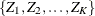has the canonical joint distribution with information levels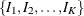for the parameter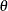(Jennison and Turnbull 2000, p. 49):

•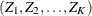is multivariate normal

•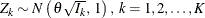•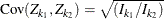,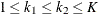In terms of the maximum likelihood estimator,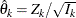,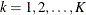, the canonical joint distribution can be expressed as follows:

•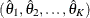is multivariate normal

•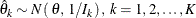•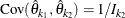,Furthermore, in terms of the score statistics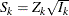,, the canonical joint distribution can be expressed as follows:

•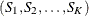is multivariate normal

•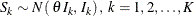•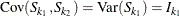,That is, the increments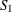,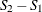, …, and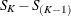are independently distributed.

If the test statistic is computed from the data that are not from a normal distribution, such as a binomial distribution, then it is assumed that the test statistic is computed from a large sample such that the statistic has an approximately normal distribution.

If the increments,, …, andare not independently distributed, then it is inappropriate to use group sequential methods in the SEQDESIGN procedure. One such example is the Gehan statistic, which is a weighted log-rank statistic for censored data. See Jennison and Turnbull (2000, pp. 232–233, 276–277) and Proschan, Lan, and Wittes (2006, pp. 150–151) for a description of statistics with nonindependent increments.

If a trial stops at an early interim stage with only a small number of responses observed, it can lead to a distrust of the statistical findings, which rely on the assumption that the sample is large (Whitehead 1997, p. 167). A group sequential design can be specified such that at the first interim analysis, there are a sufficient number of responses to ensure that the analysis to be conducted is both reliable and persuasive (Whitehead 1997, p. 167).

Alternatively, a method such as the O’Brien-Fleming method can be used to derive conservative stopping boundary values at very early stages to make the early stop less likely. That is, the trial is stopped in early stages only with overwhelming evidence.

A simple example of the group sequential tests is the test for a normal mean,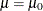. Suppose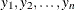are n observations of a response variable Y in a data set from a normal distribution with an unknown meanand a known variance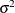. Then the maximum likelihood estimate ofis the sample mean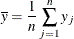The sample mean has a normal distribution with meanand variance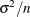: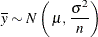An equivalent hypothesis foris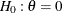, where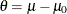. The MLE statistic for,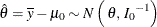where the information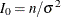.

For a group sequential test with K stages, there are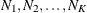observations available at these stages. At stage k, the sample mean is computed as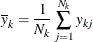where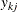is the value of the jth observation available at the kth stage and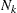is the cumulative sample size at stage k, which includes the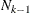observations collected at previous stages and the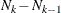observations collected at the current stage.

The maximum likelihood estimate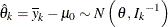where the information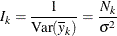is the inverse of the variance.

Thus, the standardized statistic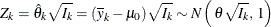The covariance of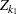and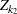,can be expressed as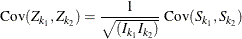where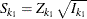and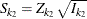.

Since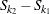is independent of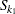,and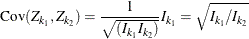Thus the statisticshas the canonical joint distribution with information levelsfor the parameter. See the section Applicable One-Sample Tests and Sample Size Computation, the section Applicable Two-Sample Tests and Sample Size Computation, and the section Applicable Regression Parameter Tests and Sample Size Computation for more examples of applicable tests in group sequential trials.